Reach Us+44-1474-556909
A Fast Analytic Simulation of Stochastic Mutation and its Application to Modeling Cancer Drug Resistance | OMICS International
Journal of Applied & Computational Mathematics
All submissions of the EM system will be redirected to Online Manuscript Submission System. Authors are requested to submit articles directly to Online Manuscript Submission System of respective journal.

# A Fast Analytic Simulation of Stochastic Mutation and its Application to Modeling Cancer Drug Resistance

Colborn JA*

Protocol Intelligence, Inc., 7667 Circulo Sequoia, Carlsbad, CA 92009, USA

*Corresponding Author:
Colborn JA
Protocol Intelligence, Inc.
7667 Ci rculo Sequoia
Carlsbad, CA 92009, USA
Tel: 7604979718
E-mail: [email protected]

Received Date: February 16, 2016; Accepted Date: March 14, 2016; Published Date: March 18, 2016

Citation: Colborn JA (2016) A Fast Analytic Simulation of Stochastic Mutation and its Application to Modeling Cancer Drug Resistance. J Appl Computat Math 5: 293. doi:10.4172/2168-9679.1000293

Copyright: © 2016 Colborn JA. This is an open-access article distributed under the terms of the Creative Commons Attribution License, which permits unrestricted use, distribution, and reproduction in any medium, provided the original author and source are credited.

Visit for more related articles at Journal of Applied & Computational Mathematics

#### Abstract

Random “Darwinian” mutation is a primary mechanism by which cancer and pathogens develop resistance to drugs, and this process has been mathematically modeled extensively. Analytic models employ simple equations and allow for very fast computation, but do not accurately predict mutation times or survival probabilities of resistant populations. Stochastic models provide a distribution of probable outcomes but involve more complex mathematics. We present here an analytic method that simulates stochastic mutation with much better accuracy than that of the standard analytic equations. This method is based on an observation that the median stochastic solution emerges at a time close to when the cumulative probability of a first mutant birth approaches unity, which can be calculated analytically. We compare our model to the median stochastic resistant population versus time for varying rates of cell division, natural death, mutation, and drug kill. Generally we find at least an order-of-magnitude reduction in the error of the birth time and the RMS normalized error relative to the standard analytic solution. This method’s speed, accuracy, and simple results make it well-suited as a tool in software and mutation models to survey the resistant heterogeneity of cancers under various treatment plans or to guide a probabilistic analysis with a stochastic model. Such models could advance progress toward a better understanding of the dynamics of resistant subpopulations, better personalized treatment plans, and longer patient survival given the complex and ever-changing sets of drugs, doses, schedules, and cancer genomics of each patient in the clinical setting.

#### Keywords

Cancer; Mutation; Cancer drug resistance; In-silico cancer modeling

#### Introduction

Random mutation is pervasive in nature and has been mathematically modeled extensively. It is a primary mechanism by which cancer and pathogens resist drugs and other systemic treatments. For example, most cancers are still incurable primarily because they develop resistance to anti-cancer drugs. This resistance arises via a variety of mechanisms [1-4], and mathematical modeling over the past four decades has improved our understanding of it [5-17]. It is now recognized that tumors are heterogeneous, resulting from random mutation of cancer-cell DNA, both before and after treatment begins, and this is an important, if not the primary, source of resistance-generating mutations in cancer [18-25]. Some mathematical models of resistance have relied on this assumption using an analytic deterministic approach and have led to elegant insights [5,9,10] when applied to limited, simple cases. Others have employed more complex mathematical stochastic machinery to produce analytical solutions to their equations [8,11-16] leading to general treatment suggestions, but these have generally addressed somewhat idealized situations and required significant computation, limiting their usefulness in the clinical setting.

For a variety of applications involving the modeling of timedependent mutation processes, computation of mutant birth times and growth rates is frequently required. Where possible, it would be useful to capture the essential features of a stochastic treatment of mutation while maintaining the simpler mathematics of an analytic approach. For modeling cancer drug resistance from a bioengineering perspective, we seek not only to better understand the biological process, but also to develop software that oncologists can use in the clinic to track and predict the evolution of drug-resistance for each cancer patient, thereby dynamically assisting with protocol decisions expected to extend patient survival. Each patient may have a unique and complex treatment history: a clinically-useful mutation model must be able to fit an arbitrary and ever-changing set of drugs, doses, schedules, and patient data. Any mutation computation may be needed dozens of times during tumor modeling, which must take only seconds to complete, typically by the oncologist on a computer or mobile device. We hypothesize that to within the accuracy of patient data, it is possible to match the median results of a stochastic mutation model with sufficient accuracy and reliability using an analytic method, and that this result can be used to computationally survey the evolution of the mutant subpopulation. We test this hypothesis here by comparing results of the two methods under a variety of input parameters. Using this result to model the simultaneous evolution of multiple mutant cancer subpopulations and comparison with patient data is in progress and will be presented elsewhere [26-28].

#### Materials and Methods

We model the mutation event from a sensitive “wild-type” cancer cell population S to a resistant “mutant” cancer cell population R under the following assumptions: cell mutation, division, and death are random Markov processes; the sensitive population is known from patient data; all cells are assumed to be cancer “stem” cells with the same probabilities of mutation, natural death, and division; and all cells divide simultaneously at the end of the cell cycle model shown in Figure 1. We model a situation in which a cancer is born and grows untreated, and treatment begins at some later time. We generate difference equations in discrete time for the sensitive and resistant populations and solve them numerically under stochastic assumptions. We then identify the average features of the stochastic result that we want to predict with an analytic approach. We then go back and generate deterministic difference equations by taking the expected value of the stochastic difference equations, solve them, and compare these solutions to the stochastic results. Finally, we adjust the analytic result based on the key observation that the median stochastic solution emerges at a time close to when the cumulative probability of a first mutant birth approaches unity.

Figure 1: The cell-cycle model prior to treatment. Each cycle starts immediately after cell division. Natural cell death occurs at the end of the G1 phase, and division occurs at the end of the cycle. All cells are assumed to be cancer “stem” cells with the same probabilities per cycle of mutation, natural death, and division of pmutate, pdie and pdivide, respectively. During treatment, drug kill of sensitive cancer cells can occur any time during the cycle prior to division.

The cycle for the sensitive cancer cell population prior to treatment is shown on the left side of Figure 1. The population is assumed to be subject to natural death at the end of the G1 phase and to division just prior to the end of each cycle, after which its value at the end of the n+1 cycle is given by

S[n+1] = S[n] – Sdie[n+1] + Sdivide[n+1]          (1)

Where Sdie is the number of sensitive cell deaths, Sdivide is the number of sensitive cell divisions, and we have neglected the effect of mutation on the un-mutated sensitive population because the mutation probability is very small. According to Figure 1, we first treat the natural death process, then division. We now analyze Equation 1 stochastically, randomly sampling the binomial distribution. We define B(N,p) as the cumulative integer obtained by randomly sampling the binomial probability distribution N times with probability p. So Equation 1 and Figure 1 yield

Ndie[n+1] = S[n]                         (2a)

Ndivide[n+1] = S[n] –B(Ndie[n+1], pdie)                                  (2b)

S[n+1] = Ndivide[n+1] + B(Ndivide[n+1], pdivide)                   (2c)

Where Ndie and Ndivide are the number of cells (trials) input into the binomial processes of cell natural death and division for each cycle, respectively, and pdie and pdivide are the probabilities of a cell naturally dying and dividing per cycle, respectively. The left-hand sides of Equations 2a-2c define the stochastic cell populations moving clockwise around the left-hand side of Figure 1 and each is input in the right-hand side of the next: Ndie[n+1] is the input population for the natural death process at the end of the G1 phase, Ndivide[n+1] is the input population for the division process at the end of the M phase, and S[n+1] is the final population at the end of the entire cycle. Combining Equations 2a-2c gives

S[n+1] = S[n] – B (S[n], pdie) + B(S[n]–B(S[n],pdie), pdivide)           (3)

We are not interested in the stochastic solutions for the sensitive population S but only want to generate an analytic expression for it that matches patient data and can be used as a mutation source for the calculation of the resistant population R[n], so we take the expected value of equation (3) to obtain

S[n+1] = S[n](1–pdie)(1+pdivide)             (4)

Which by inspection has the solution

S[n] = S0[(1–pdie)(1–pdivide)]n            (5)

and S0 = S is generally assumed to equal one. We plot S[n] in Figure 2 for example parameters of pdivide = 0.9 and pdie = 0.43 which have been selected to match the least-squares-fit of the synthetic patient data points shown in the figure. We now introduce chemotherapy treatment starting at time n = n*. Drug kill may be modeled at any single point in the cell cycle without affecting the equations. This introduces the drug kill probability per cell cycle pkill and the sensitive population during treatment becomes

S[n] = S*[(1 – pdie) (1 – pkill) (1 + pdivide)]n–n*       (6)

where S* = S0, S *= S0[(1-pdie)(1+pdivide)]n* is the sensitive population at the start of treatment. Letting a = (1-pdie) (1+pdivide) and b = a(1- pkill) gives S[n] = S0an prior to treatment and S[n] = S0anbn-n* during treatment (n ≥ n*).

Turning to the calculation of the resistant mutated population R[n] without treatment,

R[n+1] = R[n]+Rmutate[n]-Rdie[n+1]+Rdivide[n+1]      (7)

Figure 2: Solutions to the analytic difference equations without drug treatment. S[n] is the analytic solution for the sensitive, un-mutated cancer cell population.R[n] is the un-adjusted analytic solution for the resistant mutant population, which first exceeds one cell at time nx. P[n] is the probability that a first mutation has occurred, P(nmutate) = 0.9, and RA[n] is the adjusted analytic solution for the resistant mutant population

Where now we have a mutation source that adds to the population at the start of each cycle, as shown on the right-hand-side of Figure 1. Introducing the probability of mutation per cycle, pmutate, gives

R[n+1] = R[n] + B(S[n],pmutate)-B(R[n] + B(S[n],pmutate),pdie) + B(B(R[n] + B(S[n],pmutate),pdie),pdivide)           (8)

Where we have defined the stochastic cell populations moving clockwise around the right-hand side of Figure 1 and combined the resulting equations as we did for Equation 3. This equation can be solved numerically. Each solution is a “realization” in time of a possible outcome, given the probabilities. We now take the expected value of equation (8) to obtain a deterministic difference equation before treatment

R[n + 1] = (R[n] + pmutateS[n])(1-pdie)(1 + pdivide) = (R[n] + pmutateS0an)a       (9)

Which may be solved using Z-transforms to give the analytic solution

R[n] = Ran + pmutateS0an         (10)

Before treatment. Because R = 0, this gives R[n] = pmutateS0nan, the un-adjusted analytic solution for the resistant population as shown in black in Figure 2. Note a major problem with this solution is the so-called “nano-cell” problem: the solution starts immediately at time zero with a population of 10-8 cells and only exceeds a single cell at time nx. As a first approximation, we could truncate this solution prior to nx, consider the birth of the resistant population to occur at nx, and use the solution R[n] for times thereafter. However, by comparing to the stochastic results below and making a simple adjustment based on mutation probability, we will show we can improve on this approach considerably.

During treatment, the resistant population is only affected by the drug through attenuation of the mutation source term, the sensitive population, and we have

R[m + 1] = (R[m] + pmutateS0an*bm)a                   (11)

Where m = n-n*. The solution using Z-transforms again is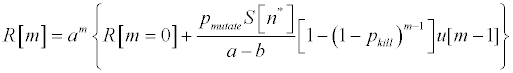(12)

Where u[m-1] is the unit step function such that u = 0 for m < 1 and u = 1 for m ≥ 1 and S[n*] = S0an* from equation (5) for our particular situation in which S[n] has been untreated since birth of the cancer. Note this solution is exponential if pkill = 0 and the effect of nonzero pkill tails off exponentially as m increases.

Equations (10) and (12) give analytic solutions for R that match the form of the median stochastic solution, but they need to be adjusted to correctly match the median mutation time of the stochastic realizations, and also to account for cases in which treatment is started early enough to limit the source of mutants and extinguish most stochastic realizations, including the median. This adjustment can be accomplished by assuming the sensitive cells do not mutate until and unless the cumulative probability P[n] of a first mutant exceeds a threshold value close to unity at time nmutate. That is, P[nmutate]≈1, where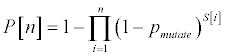(13)

We illustrate this function in Figure 2 with a threshold value of P[nmutate]=0.9 along with the adjusted resistant analytic solution RA[n], which prior to or without treatment is given by

RA[k] = (RA[k = 0]ak + S[k = 0] pmutatekak)u(k)             (14)

Where k = n-nmutate and u(k) is the unit step function. After treatment starts at time n = n* we have if n *> nmutate,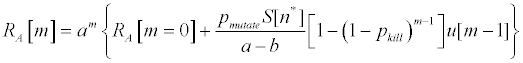(15)

Where again m = n-n*, RA[m = 0] is obtained from Equation (14), and S[n*] = S0an* from Equation (5) for our particular situation in which S[n] has been untreated since the birth of the cancer. If n*≤nmutate, then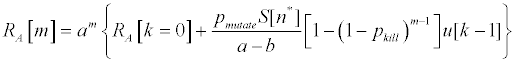(16)

and generally RA[k = 0] = 1.

#### Results

Returning to the numerical solution of Equation (8), it is useful to generate a large number of realizations of R[n] and examine their properties, in particular their median mutation times and early growth rates. We show 103 such realizations in Figure 3 along with their median at each cell cycle for the same parameters as Figure 2. We use the median to eliminate the unpredictable bias of early-born exponentially growing outliers. However, the threshold value for P[n] can be adjusted to target the stochastic mean or mode if desired. This figure shows the key features of a stochastic solution, generally most important for cell populations less than about 101 – 102 cells, that are absent in a deterministic treatment: 1) There is a range of possible outcomes for the resistant population, but in this case these tend to converge as the population increases; 2) the median mutation time is delayed relative to the deterministic solution, due to the absence of cell fractions; 3) the median date at which the stochastic solution emerges and exceeds 101 - 102 cells is further delayed due to random extinction of some realizations in the very early life of the mutant population. In the case of an exponentially growing mutation source (the sensitive population), the resistant population growth rate starts out high because the mutant source term is significant, but this term loses significance as the population grows, and the growth rate stabilizes at a lower rate driven largely by cell division. RA[n] in red, the adjusted deterministic solution, and RSM[n] in blue, the moving median of 103 stochastic realizations, lie almost on top of one another in this plot, such that RA[n] is a much better prediction of the median stochastic behavior RSM[n] than is the original analytic solution R[n].

Figure 3: Stochastic and analytic solutions to the difference equations without drug treatment. S[n],R[n], RA[n], and P[n] are the same as for Figure 2.RA[n] in red, the adjusted analytic solution, and RSM[n] in blue, the moving median of 103 stochastic realizations, lie almost on top of one another in this plot.

We systematically compare RA and RSM across a variety of input parameters, with the figures of merit being the relative error in the birth time and the root-mean-squared (RMS) normalized error. The relative error in the birth time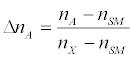(17)

And nA and nSM are the birth times of the adjusted analytic solution and the stochastic median solution, respectively. The root-meansquared (RMS) normalized error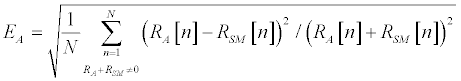(18)

We illustrate in Figure 4 for several treatment start times n* the comparison between the adjusted analytic solution RA[n] and the median stochastic solution RSM[n] along with the errors of the adjusted solution. Note that for treatment start times n*>>nmutate, well after P[n]≈1 has been achieved, the solution for RA[n] is essentially identical to that with no treatment. If treatment is started prior to nmutate, the median stochastic realization and the adjusted analytic solution for RA[n] is zero, whereas the simple analytic solution predicts mutation and exponential growth. For treatment start times close to nmutate when P[n]=0.9 the early growth rate is limited because the treatment starts killing off the source of mutants right when the resistant population needs them to avoid extinction and grow beyond a population of 101 - 102 cells. In this limited situation (Figure 4d) the median stochastic realization is close to the last-mutating realization. It therefore fluctuates very sensitively to input parameters between this solution (late mutation) and zero (no mutation), and a successful match between RA and RSM becomes less predictable. We have illustrated the analytic model predicting late mutation for this case. The result of running stochastic realizations with treatment is a bifurcation: a number of realizations result in no mutation and zero resistant population for all time, and the remainder end up growing exponentially at the rate (1-pdie)(1 + pdivide) as soon as their populations get large enough for equation (9) to apply. If treatment is delayed long enough or there is no treatment, all realizations survive and grow.

Figure 4: Stochastic and analytic solutions to the difference equations with drug treatment introduced at various timesn*, 125 realizations with pmutate =10-8, pdivide = 0.9, pdie = 0.43, and pkill = 0.4. The relative error of the birth time is ΔnA , EA is the normalized RMS error of the adjusted analytic solution (in red), and ER is that of the simple analytic solution (in black).

Figure 5 shows the effect of reducing the drug kill rate from the circumstances of Figure 4e, in which no mutation was predicted by the adjusted analytic solution. This sequence shows evolution toward the good match between the adjusted analytic solution RA and the median stochastic solution RSM as the drug kill rate is reduced, bringing the situation closer to that of no treatment such as shown in Figure 4a. Figure 5b shows an example in which the median stochastic realization is just barely mutating. This is the “worst case” scenario for matching the adjusted analytic solution RA and the median stochastic solution RSM. It occurs only in a very limited region of parameter space.

Figure 5: Stochastic and analytic solutions with drug treatment introduced at various drug kill probabilities pkill. 125 realizations with pmutate =10-8, pdivide = 0.9, pdie = 0.43 and n*= 200 other parameters the same as Figure 4e.

Figure 6 shows relative to the circumstances of Figure 4e the general insensitivity of the solution to pdivide and pdie, holding the net growth rate (1-pdie) (1 + pdivide) constant at 1.083. The principal effect is as the probabilities both decrease, the mutant extinction rate decreases for very small mutant populations owing to the lower pdie. This advances the emergence of the resistant population by a few cell cycles under the stochastic model.

Figure 6: Stochastic and deterministic solutions with various division and natural death probabilities, pdivide and pdie, while holding constant a=(1-pdie) (1 + pdivide), the net growth rate realizations with pmutate=10-8, pdie=0.43 and n*= 250, other parameters.

Figure 7 shows the effect of varying the net growth rate a = (1- pdie) (1 + pdivide) relative to Figure 4a by varying pdie while holding the division rate pdivide constant at 0.9. As shown, the accuracy of the adjusted analytic solution RA remains very good for various a. As pdie decreases and the net growth rate therefore increases, the solutions compress in time and the relative advantage of the adjusted analytic solution RA over R is diminished but remains significant.

Figure 7: Stochastic and analytic solutions without treatment, with various natural death probabilities pdie,125 realizations with other parameters the same as Figure 4a.

Figure 8 shows the effect of varying the mutation rate pmutate relative to Figure 4a. Again the accuracy of the adjusted analytic solution RA remains very good and is largely insensitive to pmutate.

We performed the calculations and generated the figures for this work on a Dell Optiplex workstation running MATLAB R2015b on an i5-2400 CPU @ 3.10 GHz running Windows 10 Pro.

Figure 8: Stochastic and analytic solutions without treatment, with various mutation probabilities pmutate,125 realizations with other parameters the same as Figure 4a.

#### Conclusion

We have presented here an analytic method that simulates stochastic mutation and applied it to modeling cancer drug resistance. This method is based on a key observation that the median stochastic solution emerges at a time close to when the cumulative probability approaches unity of a first mutant birth, which can be calculated analytically. We have compared our result to the median stochastic resistant population versus time for varying rates of cell division, natural death, mutation, and drug kill. We have found at least an order-of-magnitude accuracy improvement over the standard analytical solution under most of these conditions. This method’s speed, accuracy, and simple results make it well-suited as a tool in software and mutation models to survey the resistant heterogeneity of cancers under various treatment plans. This may be used to guide a follow-on probabilistic analysis with a stochastic model. Such models could advance progress toward a better understanding of the dynamics of resistant subpopulations, better personalized treatment plans, and longer patient survival given the complex and ever-changing sets of drugs, doses, schedules, and cancer genomics of each patient in the clinical setting. Using these results to model the simultaneous evolution of multiple mutant subpopulations and comparison with patient data is in progress and will be presented elsewhere.

#### Acknowledgements

We thank Michal Ben-Nun, Zoran Mikic, Pete Riley, and Jon A. Linker at Predictive Science Inc. for helpful discussions and comments on the manuscript.

#### References

Select your language of interest to view the total content in your interested language

### Article Usage

• Total views: 8587
• [From(publication date):
March-2016 - Sep 18, 2019]
• Breakdown by view type
• HTML page views : 8487

## Post your commentCan't read the image? click here to refresh
###### Peer Reviewed Journals

Make the best use of Scientific Research and information from our 700 + peer reviewed, Open Access Journals

### Clinical & Medical Journals

International Conferences 2019-20

Meet Inspiring Speakers and Experts at our 3000+ Global Annual Meetings

Top